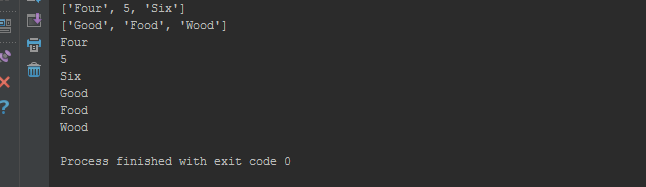## python数组_Python数组_cunchi4221的博客-程序员秘密

python数组

Python Array contains a sequence of data. In python programming, there is no exclusive array object because we can perform all the array operations using list. Today we will learn about python array and different operations we can perform on an array (list) in python. I will assume that you have the basic idea of python variables and python data types.

Python Array包含一系列数据。 在python编程中，没有排他的数组对象，因为我们可以使用list执行所有数组操作。 今天，我们将学习python数组以及可以在python中的数组（列表）上执行的不同操作。 我将假定您具有python变量和python数据类型的基本概念。

## Python数组 (Python Array)

Python supports all the array related operations through its list object. Let’s start with one-dimensional array initialization.

Python通过其list对象支持所有与数组相关的操作。 让我们从一维数组初始化开始。

### Python数组示例 (Python array example)

Python array elements are defined within the brace `[]` and they are comma separated. The following is an example of declaring python one-dimensional array.

Python数组元素在大括号`[]`中定义，并且用逗号分隔。 以下是声明python一维数组的示例。

``````arr = [ 1, 2 ,3, 4, 5]
print (arr)
print (arr)
print (arr)``````

Output of above one dimensional array example program will be:

``````[1, 2, 3, 4, 5]
3
5``````

Array indexing starts from 0. So the value of index 2 of variable arr is 3.

In some other programming languages such as Java, when we define an array we also need to define element type, so we are limited to store only that type of data in the array. For example, `int brr;` is able to store integer data only.

But python gives us the flexibility to have the different type of data in the same array. It’s cool, right? Let’s see an example.

``````student_marks = ['Akkas' , 45, 36.5]
marks = student_marks+student_marks
print(student_marks + ' has got in total = %d + %f = %f ' % (student_marks, student_marks, marks ))``````

It give the following output:

``Akkas has got in total = 45 + 36.500000 = 81.500000 marks``

In the above example you can see that, `student_marks` array have three type of data – string, int and float.

### Python多维数组 (Python multidimensional array)

Two dimensional array in python can be declared as follows.

python中的二维数组可以声明如下。

``````arr2d = [ [1,3,5] ,[2,4,6] ]
print(arr2d) # prints elements of row 0
print(arr2d) # prints elements of row 1
print(arr2d) # prints element of row = 1, column = 1``````

It will produce the following output:

``````[1, 3, 5]
[2, 4, 6]
4``````

Similarly, we can define a three-dimensional array or multidimensional array in python.

### Python阵列范例 (Python array examples)

Now that we know how to define and initialize an array in python. We will look into different operations we can perform on a python array.

### 使用for循环遍历Python数组 (Python array traversing using for loop)

We can use for loop to traverse through elements of an array. Below is a simple example of for loop to traverse through an array.

``````arrayElement = ["One", 2, 'Three' ]
for i in range(len(arrayElement)):
print(arrayElement[i])``````

Below image shows the output produced by the above array example program.

### 使用for循环遍历2D数组 (Traversing 2D-array using for loop)

The following code print the elements row-wise then the next part prints each element of the given array.

``````arrayElement2D = [ ["Four", 5, 'Six' ] , [ 'Good',  'Food' , 'Wood'] ]
for i in range(len(arrayElement2D)):
print(arrayElement2D[i])

for i in range(len(arrayElement2D)):
for j in range(len(arrayElement2D[i])):
print(arrayElement2D[i][j])``````

This will output:### Python数组追加 (Python array append)

``````arrayElement = ["One", 2, 'Three' ]
arrayElement.append('Four')
arrayElement.append('Five')
for i in range(len(arrayElement)):
print(arrayElement[i])``````

The new element Four and Five will be appended at the end of the array.

``````One
2
Three
Four
Five``````

You can also append an array to another array. The following code shows how you can do this.

``````arrayElement = ["One", 2, 'Three' ]
newArray = [ 'Four' , 'Five']
arrayElement.append(newArray);
print(arrayElement)``````
``['One', 2, 'Three', ['Four', 'Five']]``

Now our one-dimensional array arrayElement turns into a multidimensional array.

### Python数组大小 (Python array size)

We can use `len` function to determine the size of an array. Let’s look at a simple example for python array length.

``````arr = ["One", 2, 'Three' ]

arr2d = [[1,2],[1,2,3,4]]

print(len(arr))
print(len(arr2d))
print(len(arr2d))
print(len(arr2d))``````

### Python数组切片 (Python array slice)

Python provides a special way to create an array from another array using slice notation. Let’s look at some python array slice examples.

Python提供了一种特殊的方式来使用切片符号从另一个数组创建一个数组。 让我们看一些python数组切片示例。

``````arr = [1,2,3,4,5,6,7]

#python array slice

arr1 = arr[0:3] #start to index 2
print(arr1)

arr1 = arr[2:] #index 2 to end of arr
print(arr1)

arr1 = arr[:3] #start to index 2
print(arr1)

arr1 = arr[:] #copy of whole arr
print(arr1)

arr1 = arr[1:6:2] # from index 1 to index 5 with step 2
print(arr1)``````

Below image shows the python array slice example program output.

### Python数组插入 (Python array insert)

We can insert an element in the array using `insert()` function.

``````arr = [1,2,3,4,5,6,7]

arr.insert(3,10)

print(arr)``````

### Python数组弹出 (Python array pop)

We can call the pop function on the array to remove an element from the array at the specified index.

``````arr = [1,2,3,4,5,6,7]

arr.insert(3,10)
print(arr)

arr.pop(3)
print(arr)``````

That’s all about python array and different operations we can perform for the arrays in python.

python数组

### Ceph学习(1)---Ceph入门_一百零八步的博客-程序员秘密_ceph教程

Ceph学习(1)---Ceph入门

### 频繁项集挖掘算法在告警关联中的应用_云智慧AIOps社区的博客-程序员秘密_频繁项挖掘

# 技术黑板报 # 第十期推荐阅读时长：15min在上一篇技术黑板报中，我们介绍了频繁项集挖掘这一问题，并讲解了Apriori算法与FP-Growth算法的技术原理。本期技术黑板报我们将主要围绕频繁项集挖掘算法的实际应用，即当该算法应用到告警关联场景中时，我们遇到了哪些问题，如何解决这些问题，以及我们如何在原始FP-Growth算法的基础上进行改进，从而研发了专用于告警关联场景下的CW-FP-Growth算法。为了展示该算法的实际效果，我们在文末给出了这一算法在脱敏数据中的案例。..

### js中如何验证一个字符是否为大写字母,不用正则表达式_wyd1022的博客-程序员秘密_js判断大写字母

unction allCaps(text){ for(var i=0;i&amp;lt;text.length;i++){ var c=text.charAt(i); if(c&amp;lt;'A' || c&amp;gt;'Z') return false; } return true;}